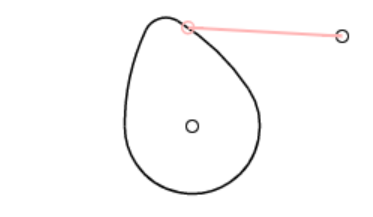##### Cam and Follower

I was able to get a functioning cam simulation running in JavaScript. It was a little tricky and isn’t quite perfect yet. Mostly, I had to remember how my code works with the HTML5 canvas and with the coordinate system that I use for the geometric constructions page.

The cam is composed of four arcs. The center of the cam rotation is a fixed point on the page. The length of the cam from the  center to the nose, as well as the radii of the nose, the base, and the tangents, are all hard-coded. The locations of the arcs are calculated from that information.

From the arcs and the circle that defines all possible locations of the follower, the code simply figured out where on each arc the follower circle intersects. There are theoretically eight possible points, but in reality and with the shape of the cam and location of the follower, there are never that many. Then the code simply ”knows” that the follower pivot is to the right making the up-most point of intersection obviously the one I want.

The Math used for this includes a circle-to-circle intersection function that I’ve had around for years, and some calls to the arctangent function to get the angles of various points relative to various other points. Arcs are the only difficult data to deal with because they have have clockwise or counterclockwise directions between their start and end points. I use start and end points instead of angles because all of the arcs are calculated using intersection functions that return points. Using angles would increase the calculations use to construct the arcs but would reduce the calculations later to find if intersection points lay on an arc or off of the arc. the canvas uses angles for drawing arcs so I should have used angles instead of points. Oh well.

Next will be the same type of simulation but for a cam defined by Bezier curves. That’s going to be a bug challenge!